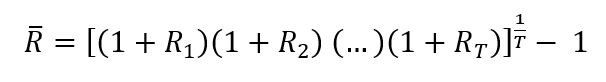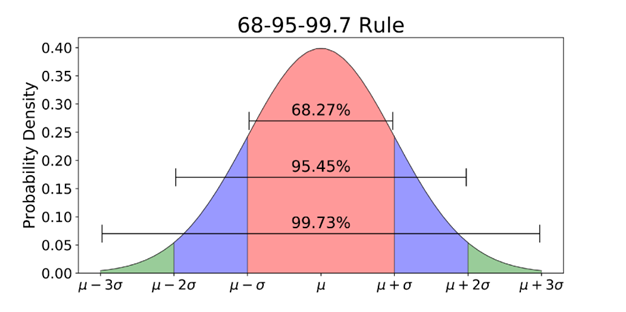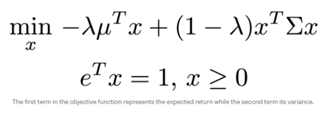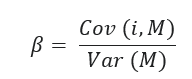top of page
Search

# Overview on Portfolio Management

Updated: Nov 30, 2021

“A good portfolio is more than a long list of good stocks and bonds. It is a balanced whole, providing the investor with protections and opportunities with respect to a wide range of contingencies.”

Harry Markowitz

## Introduction

Portfolio management is the strategy that each investor needs to plan based on their strategic business objectives. It must take into account goals, tolerated risk exposure and time frame. The main challenge is to find the appropriate balance between the expected return of the portfolio and the risk associated with it, which are positively correlated as stated by the risk-return trade-off principle: higher returns always imply higher risk.

This paper gives a broad view of the different phases of portfolio management, starting from the planning step, whose aim is to understand the investor’s need, and going on with the execution step, made of asset allocation, security selection and portfolio construction. Last but not least the evaluation step consists on rebalancing the portfolio. Despite all phases are described, the focus of the article is on asset allocation with an in-depth on the Modern Portfolio Theory.

## Planning step

### Active VS passive investors

There are two types of investment styles: active and passive. Passive investors buy assets that mirror a particular market index and hold them in the long-term without changing the composition of the portfolio and not considering the daily fluctuations in prices. The assumption behind passive management is the efficient market hypothesis: the idea that the share price reflects all available information which implies that in the long run it is impossible to beat the market.

Portfolio management instead exists thanks to active investors: they believe they can outperform the market by selecting an optimal portfolio that can provide a higher average return than the market. The main downturn of active management is that to be profitable you need to earn a return high enough to cover the many costs, like management fees and the transaction costs of trading.

### The Investment policy statement

Portfolio planning means studying a strategy to construct a portfolio expected to satisfy the investor’s investment objectives. The investor policy statement is a written document drafted by portfolio managers to outline and understand the investors’ features and expectation. For this reason it must:

· Describe the overall investment objective;

· State the return, distribution and risk requirements;

· Define the risk tolerance of the investor

· Describe relative constraints

· Describe other considerations relevant to the investment strategy

### Risk aversion

One of the most important aspects investors must take into account in building their portfolio is their degree of risk aversion. Risk aversion is the tendency to prefer low-uncertainty settings over high-uncertainty ones despite the possibility of higher return:

• very risk-averse investors are conservative and choose to safeguard the liquidity and safety of their investment over the opportunity of higher reward. Their portfolios are more liquid and do not contain volatile assets, and the risk is close to 0. Safer, low risk investments are government bonds, certificates of deposits, treasury securities, life Insurance, Investment Grade Corporate Bonds.

• risk-neutral investors do not take risk into account when making an investment choice: their decision is only based on potential gains

• less risk-averse investors are willing to take on more risk with the expectation of a higher reward

In investing, the risk lies in the volatility of the portfolio, associated with the standard deviation of the returns of the assets in the portfolio.

## Execution step

The chapter begins with a brief overview of some elements essential to understand portfolio theory.

## Standard deviation as measure of risk

Standard deviation is a measure of how much an investment's returns can vary from its average return. The average return can be computed:

• arithmetically as the mean return:• geometrically using the formula of the holding period return:Standard deviation measures the dispersion of data with respect to the average return: a high value imply that the return could either be much higher or much lower than the average. This means increased uncertainty and therefore risk.The figure shows that there is a 99,73% probability that the return will fall on the range , meaning that the lower is the standard deviation, the smaller is the range, the smaller are the possible losses and this reduces uncertainty.

Algebraically, standard deviation is measured as the square root of variance, which in turn is computed using the distance of each return from the average return:## Diversification

Diversification is a way to reduce the risk of a portfolio. The core idea is that different assets are likely to move in different directions with respect to the market movements: gathering similar assets increases risk because they move in the same direction, so both returns and losses are emphasized. Instead by combining different assets that go in autonomous directions, in case of bad performance of an asset, another one will make up for losses. Uncertainty and therefore risk are reduced, but returns as well because it is very unlikely that all stocks perform well at the same moment.

## Covariance and correlation

In portfolio theory, the indicator used to express this concept is covariance, which measures the relationship between two random variables. It evaluates to what extent the variables change together:• Positive covariance: Indicates that two variables tend to move in the same direction.

• Negative covariance: Indicates that two variables tend to move in inverse directions

For example, let’s take into consideration a portfolio with two stocks, X and Y. Covariance is measured on the return of both stocks for one week. Point 1 shows the return levels of the first day. If the second day the market performs better and X’s returns are higher and Y’s returns are higher and this happens for all days, the final shape on the Cartesian plane will look like an upward sloping line: the stocks have positive correlation. If we use a bigger data set, the result will be a cloud of points with an upward sloping direction.The opposite will happen with negative covariance, where the cloud is downward sloping. If there is no correlation between the two stocks, it is impossible to tell the direction of the cloud.Studying covariance we can only tell something about the relation between the assets, this is why we need correlation to compute the degree of strength of this relationship, either positive or negative:As long as the correlation between the two stocks is less than 1, diversification is a way to reduce the overall risk of the portfolio, because the stocks do not move in the exact same direction. If correlation is 1 it means that the risk of holding a portfolio with two assets is the same as the sum of the risk of holding the assets separately. In all other cases, holding a portfolio is less risky because returns and losses offset each other, making the portfolio return less volatile.

By drawing the lines for each level of correlation, we can easily see how for the same return level, the points with lower correlation are less risky.## The Markowitz model

The Markowitz model is a portfolio optimization model and develops around the idea that a rational investor:

• given two portfolios with equal risk, he/she would prefer the one with higher return.

• given two portfolios with equal return, he/she would prefer the one with lower risk.

So instead of choosing between maximising gains or minimising variance, we can combine both and seek to minimise this equation:• "x" is the proportion of money invested in each asset, where the sum of all x is 1.

• "Lambda" is the risk-return preference level.

The aim is to solve for the optimum x* vector, for various values of lambda, which is the optimum portfolio for each risk-return preference.

It is then possible to compute the expected return and standard deviation values and to draw the true efficient frontier which suggests the most efficient portfolio for every risk-return level.• Choosing a portfolio under the efficient frontier is inefficient because it means it is possible to build a portfolio with the same standard deviation (x), but with higher return (y1>y).

• The extreme point to the right is called minimum variance portfolio and it is the least risky portfolio, associated with the lowest expected return.

## The Modern Portfolio Theory

MPT states that investors should select a portfolio from the efficient set, depending on their risk appetite. The risk associated with each portfolio depends on the degree of diversification, the extent to which the assets in it move in an autonomous way.

MPT lies on two strong assumptions, which are the base for most of its critics: the first is that markets are efficient and the second is that the correlation between assets is fixed whereas in real world it is not constant.

### Asset allocation

We have now understood how to find the optimal portfolio for each level of risk aversion and drawn the efficient frontier. Asset allocation deals with finding the proper combination between the risky portfolio and the risk-free assets, which makes the investment opportunity much richer. Risk-free assets’ feature is a known future return and this is why they hold a minimum degree of uncertainty and risk. The most common example is that of assets backed by governments, because they are expected to reach maturity, like the debt obligations issued by the U.S. Department of the Treasury (bonds, notes, and especially Treasury bills). Because they are so safe, the return on risk-free assets is very close to the current interest rate. Nevertheless if the country has a higher risk of default , the government bond might not necessarily be considered risk-free.To graphically display the risk-free asset, we must draw a straight line in the efficient set graph, connecting the risk-free rate to the portfolio chosen by each investor, like points P and S.

When adding a risk-free asset to the risky portfolio (ex.: portfolio S+risk-free asset), the risk is reduced because its low volatility contributes to reduce the variance of the whole portfolio. Lower risk means automatically lower return, in fact X>X1 but also Y>Y1. Each investor can decide which proportion of his asset to be risky and risk-free by moving along the straight line, which is called Capital allocation line.

The interesting fact is that the portfolio that combines S + risk free asset in the picture gives a higher return than portfolio P or any other portfolio, given the same risk level. This means that the combination of portfolio S with the risk free rate is always the optimal solution: even if an investor would like to reduce the riskiness of portfolio S, it is more profitable to do so by combining it with a risk-free asset instead of choosing another point in the efficient frontier.

In general, we can say that the portfolio that respect the tangency condition with one of the capital allocation lines is the optimal risky portfolio, and the line takes the name of Capital market line.

In the scenario of homogeneous expectations, the optimal risky portfolio is the market portfolio, but this would happen only under the very strong assumption of market efficiency, namely:

• All investors have the same information

• All investors process information in the same way

This means all investors would draw the same efficient frontier and would find the same tangency point, which would be the market portfolio.

In reality instead all investor process information in different ways and this is why all portfolios are different, which leads us to the topic of security selection.

## Security selection

This is the phase of selection of the stocks that will form the portfolio. When considering a stock investors should make a fundamental analysis of the sector of the security studying indicators such as price-earnings (P/E), price-book (P/B), price-cash flow (P/C), price-sales (P/S), and revenue and earnings growth, which can be tracked with the sector Exchange traded fund (ETF).

Furthermore it is important to evaluate the risk carried by each security, because it will contribute to the risk of the portfolio and to evaluate if it is systematic or unsystematic.

## Idiosyncratic/unsystematic risk

It is the risk specific to each asset. Possibility that the stock price will experience fluctuations. It is a company-specific risk, and can depend on factors like the entry of new competitor in the market, the launching of new products, an organizational redesign, change in management and so on. Unsystematic risk is foreseeable and can be offset by diversification.

## Systematic risk

It refers to the risk that is common to the entire market. It is caused by factors beyond the control of a specific company or individual, it is external to the organization. Since all assets carry this risk, it is not possible to eliminate it through diversification. This is why is also called non-diversifiable. It is made of four types of risks:

• Market risk: tendency of investors to follow the direction of the market.

• Interest rate risk: changes in the price of a security due to changes in interest rate

• Purchasing power risk : in case of positive inflation, if the income does not grow proportionally, the investor will experience less purchasing power.

• Exchange rate risk: uncertainty associated with changes in the value of foreign currencies

Using diversification investors can reduce the idiosyncratic risk, but not the systematic risk, meaning there is a minimum amount of risk that all investors have to bear, intrinsic in the market.## Beta as measure of systematic risk

Beta is the indicator used to represent the systematic risk. It measures the movements of an asset or portfolio according to movements of the overall market portfolio, thus it is an indicator of volatility. In other words, the beta of each stock represents its contribution to the non-diversifiable risk of the market portfolio.## The Capital Asset Pricing Model

When taking investment decisions, investors are willing to take on more risk than the risk-free rate only if they are compensated by a higher level of return, called risk premium. According to the risk aversion of each investor, they will choose the appropriate degree of risk and calculate the level of expected return through the CAPM formula. Therefore the Capital Asset Pricing Model describes the relationship between systematic risk and expected return for assets, the latter used to discount the expected dividends and capital appreciation of the stock over the expected holding period.

• The risk-free rate in the CAPM formula accounts for the time value of money.

• The beta represent the contribution of the stock to the systematic risk of the market. If a stock is riskier than the market, it will have a beta greater than one and since there is a positive relation between and , a riskier stock implies higher expected returns.

• The factor is the market risk premium, the additional return an investor will receive (or expects to receive) from holding a risky market portfolio instead of risk-free assets.

The security market line is the graphical representation of the CAPM model: it is an up-ward sloping curve, with β as slope and the risk-free rate as intercept, the minimum return an investor can obtain, correspondent to 0 risk level.The stocks not lying on the curve are overvalued or undervalued so they are not correctly priced by the market. During security selection, investors try to spot undervalued stock to buy and instead avoid overvalued stock, expected to lose price while moving closer to their real value.

## Evaluating step

### Rebalancing

Rebalancing is the process of adjusting the allocation of assets in the portfolio in order to keep it in line with the investor’s preference, risk tolerance and constraints.

The value of different assets in the portfolio will change during time, and this is why investors need to regularly rebalance the weights of their investment to maintain the desired proportion between risky and risk-free assets.

For example, the portfolio of a retiree that starts out with a 75% in risk-free assets and 25% in risky stocks could, after an extended market rally, shift to an 50-50 allocation. The investor has made a good profit, but there are two problems: the portfolio is now exposed to an undesirable level or risk and secondly the portfolio manager may not have the appropriate expertise to manage a portfolio very different from the one he originally designed..

There are two classes of rebalancing:

• Constant mix strategy: the weight of the holdings do not change

1. Calendar rebalancing: adjusting to the original allocation at a desired frequency.

2. Percentage of portfolio rebalancing: every asset has a target and a range in which its value can oscillate (ex.: ). The portfolio is rebalanced every time an asset jumps outside of the allowable band. The degree of volatility allowed (band size) is determined according to the transaction costs of rebalancing (more costs, wider band), the price volatility of the asset (high volatility, high risk, narrow band) and the correlation with the other holdings (high correlation, wider band).

• Constant proportion portfolio insurance. Assumption that as the value of the portfolio increases, also the risk tolerance of the investor will become higher, and a higher percentage will be invested in risky asset. Nevertheless this works only if there is a safety reserve held in cash or risk-free assets.

## ConclusionPortfolio management is the process designed to build an optimal portfolio for each active investor. On one side the portfolio must be able to outperform the market and assure a higher profit than the market portfolio or an index, on the other side it has to take into consideration the degree of risk aversion of the investor. This is why portfolio manager use to write the Investment Policy Statement as first step of the process, to make clear which are the targeted return, the risk tolerance and the constraints of each investor.

Through portfolio theory, it is then possible to balance risk and return of the portfolio, studying the correlation between stocks and trying to eliminate the systematic risk of assets trough diversification. The result is the efficient frontier: the set of efficient portfolios according to the degree of risk aversion of each investor.

The next step is to identify which percentage of the budget to invest in risky assets and in risk-free ones, called asset allocation. The portfolio manager than proceeds with stock selection: the Capital Asset Pricing Model is one of the ways stocks are priced and can be useful to spot undervalued stocks once the security market line is drawn.

The job of the portfolio manager does not finished once the portfolio is built: the value of the assets in it will change over time, causing variation in the proportion of risky and riskless assets and modifying the risk degree carried by the portfolio. This is why rebalancing is an intrinsic part of the process and must be carried out thoroughly.

SourcesConnect with Chiara Dal Monte on Linkedin